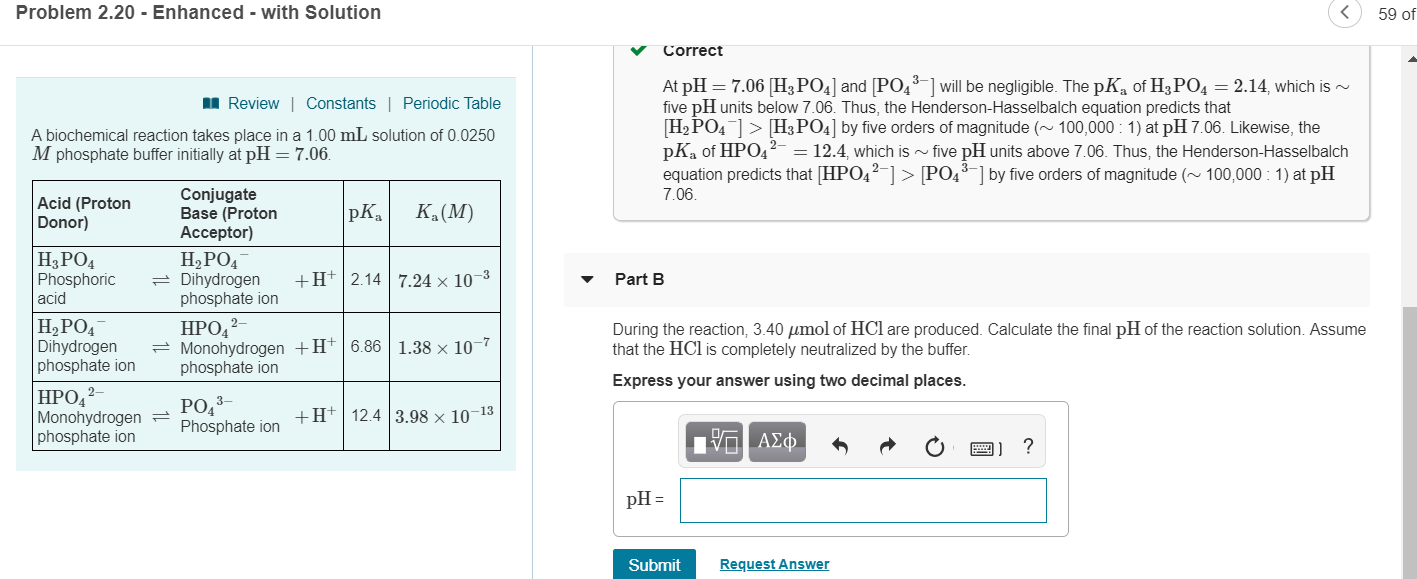# Problem 2.20 -Enhanced - with Solution 59 of Correct At pH 7.06 H PO4] and[PO will be negligible. The pKa of H3 PO 2.14, which is five pH units below 7.06. Thus, the Henderson-Hasselbalch equation predicts that H2 PO4H3PO by five orders of magnitude(100,000: 1) at pH 7.06. Likewise, the pKa of HPO4212.4, which is~five pH units above 7.06. Thus, the Henderson-Hasselbalch equation predicts that HPOP43by five orders of magnitude(100,000: 1) at pH 7.06 Review| Constants | Periodic Table A biochemical reaction takes place in a 1.00 mL solution of 0.0250 M phosphate buffer initially at pH 7.06 Acid (Proton Donor) Conjugate Base (Proton Acceptor) pKaKa(M) H3PO4 PhosphoricDihydrogen H+2.14 7.24 x 103 acid H2PO4 phosphate ion HPO2 phosphate ion PO4 3- Part B H2PO4 DihydrogenMonohydrogen +H 6.86 1.38 x 10-7 phosphate ion During the reaction, 3.40 μmol of HCl are produced. Calculate the final pH of the reaction solution. Assume that the HCl is completely neutralized by the buffer. Express your answer using two decimal places HPO2 Monohydrogen Phosphate ion phosphate ion +H 12.4 3.98 x 10-13 pH- Submit Request Answer

Question

Part B of that question in the image. Please, thank you.help_outlineImage TranscriptioncloseProblem 2.20 -Enhanced - with Solution 59 of Correct At pH 7.06 H PO4] and[PO will be negligible. The pKa of H3 PO 2.14, which is five pH units below 7.06. Thus, the Henderson-Hasselbalch equation predicts that H2 PO4H3PO by five orders of magnitude(100,000: 1) at pH 7.06. Likewise, the pKa of HPO4212.4, which is~five pH units above 7.06. Thus, the Henderson-Hasselbalch equation predicts that HPOP43by five orders of magnitude(100,000: 1) at pH 7.06 Review| Constants | Periodic Table A biochemical reaction takes place in a 1.00 mL solution of 0.0250 M phosphate buffer initially at pH 7.06 Acid (Proton Donor) Conjugate Base (Proton Acceptor) pKaKa(M) H3PO4 PhosphoricDihydrogen H+2.14 7.24 x 103 acid H2PO4 phosphate ion HPO2 phosphate ion PO4 3- Part B H2PO4 DihydrogenMonohydrogen +H 6.86 1.38 x 10-7 phosphate ion During the reaction, 3.40 μmol of HCl are produced. Calculate the final pH of the reaction solution. Assume that the HCl is completely neutralized by the buffer. Express your answer using two decimal places HPO2 Monohydrogen Phosphate ion phosphate ion +H 12.4 3.98 x 10-13 pH- Submit Request Answer fullscreen